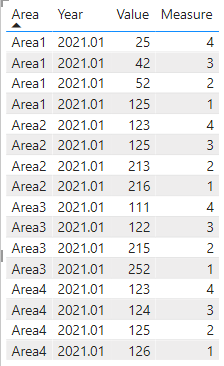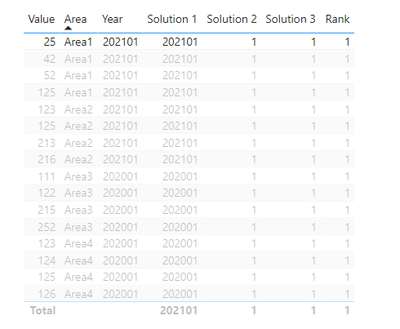cancel
Showing results for
Did you mean:

Fabric is Generally Available. Browse Fabric Presentations. Work towards your Fabric certification with the Cloud Skills Challenge.New Member

## Top 3 Value for each category and year

Hi im trying to create a Top 3 measure (rank) for each Year and Area. Is it possible to create a measure that rank the value based on dimension value area and year like:I have already tried this post, and cant get it to work in my scenario:

https://www.sqlbi.com/articles/filtering-the-top-3-products-for-each-category-in-power-bi/

1 ACCEPTED SOLUTIONCommunity Support

Here are the steps you can follow：

1. Create measure.

``````rank =
RANKX(
FILTER(ALL('Table'),'Table'[Area]=MAX('Table'[Area])&&'Table'[Year]=MAX('Table'[Year])),
CALCULATE(SUM('Table'[Value])),,DESC)``````

2. Result:Best Regards,

Liu Yang

If this post helps, then please consider Accept it as the solution to help the other members find it more quickly

7 REPLIES 7Anonymous
Not applicable

Did You Try This Measure?
Rank within Area =

RANKX (
ALLEXCEPT ( Table1, Table1[Area] ),
CALCULATE ( SUM ( Table1[Value] ) )
)Community Support

Here are the steps you can follow：

1. Create measure.

``````rank =
RANKX(
FILTER(ALL('Table'),'Table'[Area]=MAX('Table'[Area])&&'Table'[Year]=MAX('Table'[Year])),
CALCULATE(SUM('Table'[Value])),,DESC)``````

2. Result:Best Regards,

Liu Yang

If this post helps, then please consider Accept it as the solution to help the other members find it more quicklyAnonymous
Not applicable

Hi, @Wanderer1985  Please Try This Measure.

``````Rankx =
RANKX (
FILTER (
ALL ( 'Table '[Area], 'Table '[Year] ),
'Table'[Area] = MAX ( 'Table'[Area] )
),
CALCULATE ( SUM ( 'Table'[Value] ) )
)``````New Member

Hi, this also only gives 1 in return...Anonymous
Not applicable

ok, Try This Measure.

``````Rank within Area =
RANKX (
ALLEXCEPT ( Table1, Table1[Area] ),
CALCULATE ( SUM ( Table1[Value] ) )
)``````

I Try This Measure in your file and it's working. if your got a solution please mark this as a solutionSuper User

@Wanderer1985 , Create a simple TOP 3 and use that

Top 3= calculate(max(Table[Year]),TOPN(3,allselected(table[Year]),calculate(sum(Table[Value])),DESC), values(table[Year]))

or create a rank measure and filter at visual level for <=3
Rankx(allselected(table[Year]), calculate(sum(Table[Value])),,desc)

or

Rankx(filter(summarize(allselected(table),Table[Year], table[Area]), [Area] =max([Area])), calculate(sum(Table[Value])),,desc)New Member

Hi amitchandak.

I have tried all 3 solutions now - and do not get the expected result:I have added the PBIX file with the example if you would like to have a look at the scenario 🙂Announcements#### Power BI Monthly Update - November 2023

Check out the November 2023 Power BI update to learn about new features.#### Fabric Community News unified experience

Read the latest Fabric Community announcements, including updates on Power BI, Synapse, Data Factory and Data Activator.#### The largest Power BI and Fabric virtual conference

130+ sessions, 130+ speakers, Product managers, MVPs, and experts. All about Power BI and Fabric. Attend online or watch the recordings.Top Solution Authors
Top Kudoed Authors
Users online (2,010)